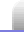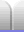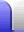# Factorising Algebra

Factorisation doesn't just work with numbers; it can be used with algebra as well. With this example we find common factors (one step at a time). First the coefficient, then the variable:

 48x2 – 3x6 = 3(16x2 – x6) = 3x2(16 – x4)

So the original expression 12x2 – 3x6 has factors of 3x2 and 16 – x4. Now, 16 – x4 is in a pattern that we should recognise (the difference of two squares), and can also be factorised. The two factors will be very similar, but with a difference of sign for the x terms. It's easier to see this going in reverse – a process called expanding – with an extra line of working shown in between, which we'll include here. Note that the x2 terms cancel each other out.

 16 - x4 = 16 - 4x2 + 4x2 – x4 = (4 + x2)(4 – x2)

Notice that 4 – x2 is in the same pattern, so we have another factor which can itself be factorised.

 4 – x2 = 4 – 2x + 2x – x2 = (2 + x)(2 – x)

And so we finally have our answer. It doesn't matter which order the factors are written in (because multiplication is commutative). The 3x2 could be put within its own brackets, but it normally is not.

 48x2 – 3x6 = 3(16x2 – x6) = 3x2(16 – x4) = 3x2(4 + x2)(4 – x2) = 3x2(4 + x2)(2 + x)(2 – x)

So the factors of 48x2 – 3x6 are 3x2, 4 + x2, 2 + x, and 2 – x.Home Astronomy Chemistry Electronics & Computers Mathematics Physics Field Trips Turn on javascript for email link